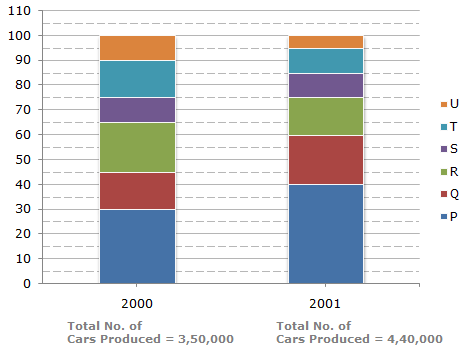# Data Interpretation - Bar Charts - Discussion

Discussion Forum : Bar Charts - Bar Chart 9 (Q.No. 4)
Directions to Solve

The bar graph given below shows the percentage distribution of the total production of a car manufacturing company into various models over two years.

Percentage of Six different types of Cars manufactured by a Company over Two Years4.
If 85% of the S type cars produced in each year were sold by the company, how many S type cars remain unsold?
7650
9350
11,850
12,250
Explanation:

Number of S type cars which remained unsold in 2000 = 15% of 35,000

and number of S type cars which remained unsold in 2001 = 15% of 44,000.Total number of S type cars which remained unsold

= 15% of (35,000 + 44,000)

= 15% of 79,000

= 11,850.

Discussion:
16 comments Page 1 of 2.

Wangmo Tamang said:   1 year ago
1. Total car produced in 2000 and 2001 as shown in the graphs:
2000: 10 % of 350,000= 35,000.
2001: 10 % of 440,000= 44,000.
Total car produced in both the year: 35,000+44,000=79,000,

2. Total car sold: 85 %.
Therefore, 85 % of 79,000.
85/100*79,000= 67,150.

3. Unsold car:
79,000- 67,150= 11,850.
That is the remaining 15 % ( 100- 85).

Samdrup pelmo said:   3 years ago
2000 = 10% of 35,0000=35000.

2001 = 10% of 44,0000=44000.

Therefore the Total number of S type cars which remained unsold;
100%-85%=15%.

15% of 35000=5250
15% of 44000=6600
5250+6600 = 11,850.

Bagir said:   3 years ago
Agree @Prateek.

I think it's 350000 instead of 35000.
Same as 440000 instead of 44000.
15% of 350000 = 52500
15% of 440000 = 66000
52500 + 66000 = 118500 (instead of 11850).

Nilekani Nandan said:   4 years ago
= 15% (10% of 350k + 10% of 440k).
= 15% (790),
= 11850.

Shubham said:   5 years ago
@All.

It is given that 85% is sold, first take out how many cars are produced/manufactured so 10%of 3,50,000 is done. Now we got that 35,000 are manufacture, then it is said that 85% is sold out of 35000 therefore 15% is not sold. That's why we are taking 15% of 35,000 same in 2001.

Hope you all understand.

Chidera said:   5 years ago
Still don't get. Why it is 15% of 35000 instead of 15%/of 350,000 and vice versa?

Riri said:   5 years ago
Exactly as 10% shown in bar graph.

Apoorv said:   7 years ago
For Unsold cars.

15% (10% of 3,50,000) + 15% (10% of 4,40,000)

Hope you get it guys.

Bappi said:   7 years ago
If I take 85% of 3,50,000 so I got = 3,50,000 - 297500 = 52500 which is 15%. So anyone just tells me how is it possible rest value?

Prateek said:   7 years ago
Why is not multiplied by 350000 and 440000 respectively in above question?